Latest Banking jobs   »   Quantitative Aptitude Quiz For IBPS PO...

# Quantitative Aptitude Quiz For IBPS PO Mains 2022- 4th January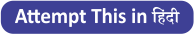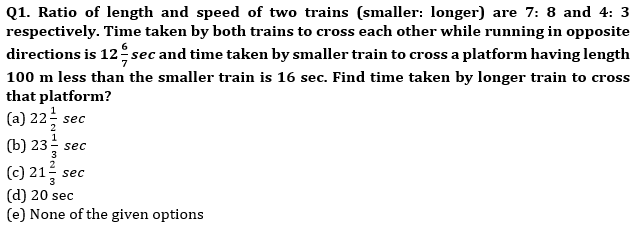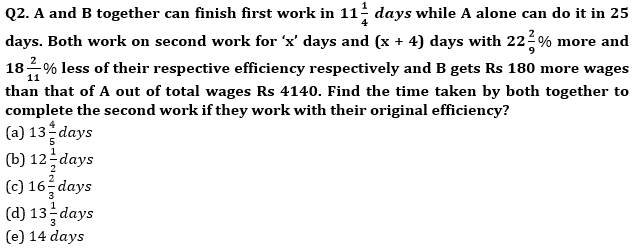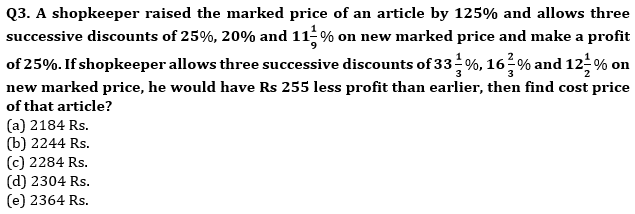Q4. Bag A contains ‘P’ green and 18 yellow balls while bag B contains ‘(P + 2)’ green balls and 22 more number of yellow balls than that of in bag A. Probability of selecting a green ball from bag A is 1/12 more than probability of selecting a green ball from bag B. Find total number of balls in bag B. (P<50)
(a) 48
(b) 54
(c) 60
(d) 84
(e) 66

Q5. Ratio of speed of boat P and Q in still water is 2 : 3. If time taken by boat ‘Q’ to cover a certain distance in upstream is equal to that of ‘P’ to cover 60% of that distance in upstream and difference of the distance covered by ‘Q’ and ‘P’ in 5hr in downstream is 60km then find ratio of speed of boat ‘P’ in downstream to ‘Q’ in upstream?
(a) 3 : 2
(b) 1 : 2
(c) 1 : 1
(d) 4 : 5
(e) 5 : 4

Q6. A mixture contains milk and water in the ratio of 9 : 2. 44 lit of mixture is taken out and 12 lit of water is added to it, such that ratio of milk to water becomes 3:1. Now another mixture of 64 lit having milk and water in ratio of 3:5 is added to it. Find ratio of milk to water in the final mixture?
(a) 32 : 17
(b) 34 : 19
(c) 7 : 4
(d) 24 : 11
(e) 33 : 19

Q7. Ratio of present age of Simmi & Rimmi is 4 : 3 while ratio of age of Simmi 6 years later to present age of Rina is 3 : 1. Ratio of present age of Rimmi to present age of Rina is 2 : 1. Find average of present age of all the three (in years).
(a) 37
(b) 36
(c) 32
(d) 34
(e) 38

Q8. A shopkeeper sells an article at Rs. 720 by making a profit of x% and on interchanging selling price with cost price, it would have a loss of y%. Find the selling price if an article was sold at y% profit whose cost price is Rs.720 (given x : y = 9 : 7)
(a) Rs. 810
(b) Rs. 800
(c) Rs. 1100
(d) Rs. 880
(e) Rs. 660

Q9. There are two rectangular fields of same area. The length of first rectangular field is a% less than the length of the second field and breadth of the first field is (4a)% greater than the breadth of the second field. Find the value of ‘a’ if a is non-zero number.
(a) 60
(b) 75
(c) 80
(d) 90
(e) None of these

Q10. A sum is divided between Aman and Vikash in the ratio of 3 : 5. Aman purchased a motorbike from his money which depreciates 20% per annum while Vikash invested his amount in a scheme which offers compound interest at 20% per annum. By what percentage, the total sum will change after two years?
(a) 12%
(b) 14%
(c) 16%
(d) 18%
(e) 20%

Solutions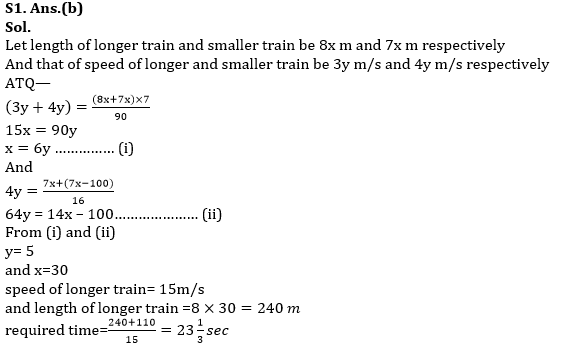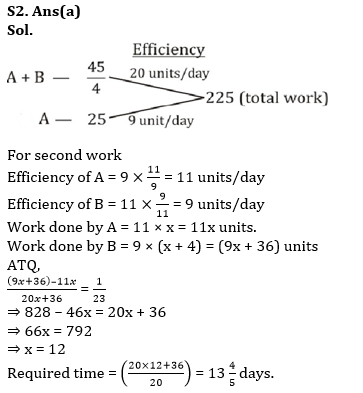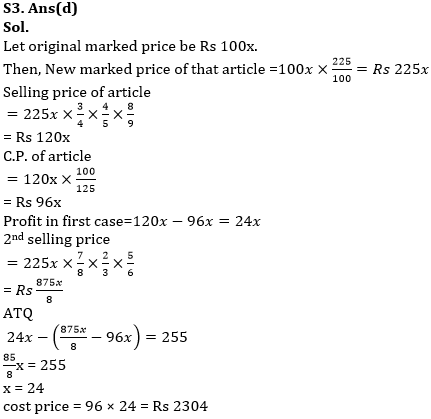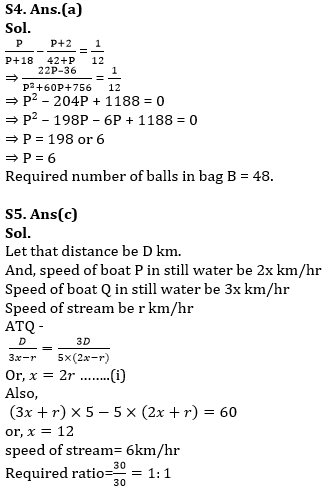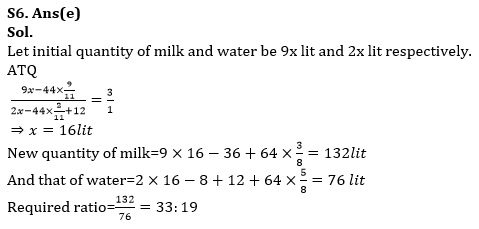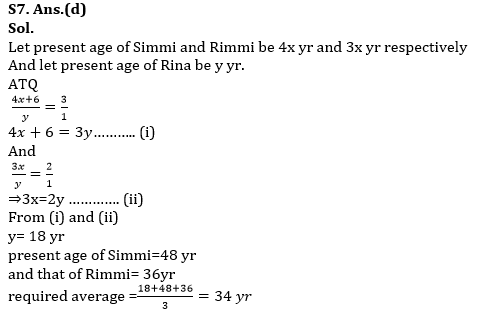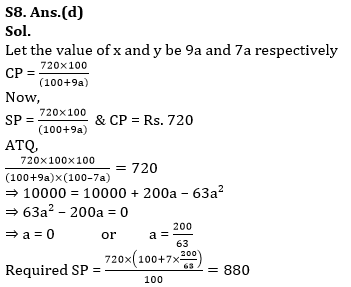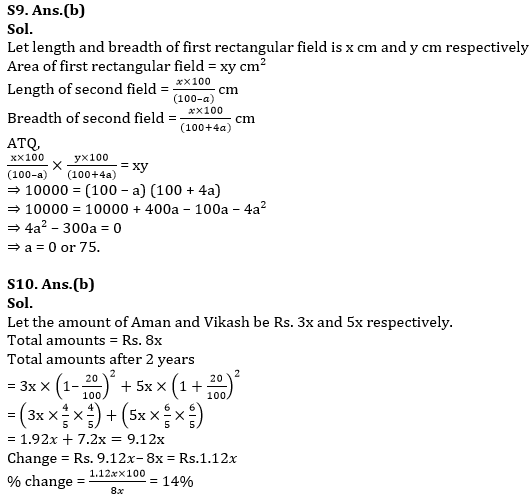#### Congratulations!Union Budget 2023-24: Free PDF